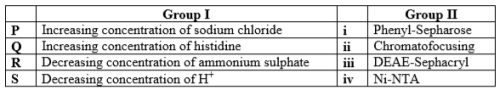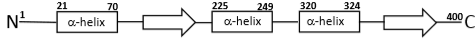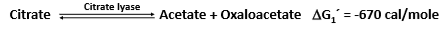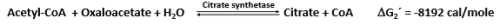# Life Sciences - (XL) 2018 GATE Paper (Practice Test - 2)

## 20 Questions MCQ Test GATE Past Year Papers for Practice (All Branches) | Life Sciences - (XL) 2018 GATE Paper (Practice Test - 2)

Description
Attempt Life Sciences - (XL) 2018 GATE Paper (Practice Test - 2) | 20 questions in 30 minutes | Mock test for GATE preparation | Free important questions MCQ to study GATE Past Year Papers for Practice (All Branches) for GATE Exam | Download free PDF with solutions
QUESTION: 1

Solution:
QUESTION: 2

Solution:
QUESTION: 3

### Which one of the following conformations of glucose is most stable?

Solution:
QUESTION: 4

Which one of the following profiles represent the phenomenon of cooperativity?

Solution:
QUESTION: 5

Which one of the following amino acids is responsible for the intrinsic fluorescence of proteins?

Solution:
QUESTION: 6

The glycosylation of the proteins occurs in_____.

Solution:
QUESTION: 7

Which one of the following properties of the myeloma cells is used in the hybridoma technology to generate monoclonal antibody?

Solution:
QUESTION: 8

The movement of protons through the FoF1-ATPase during mitochondrial respiration is required for ____

Solution:
QUESTION: 9

The number of NADP+ molecules required to completely oxidize one molecule of glucose to CO2 through pentose phosphate pathway is ____ (correct to integer number).

Solution:
QUESTION: 10

Measurement of the absorbance of a solution containing NADH in a path length of 1cm cuvette at 340 nm shows the value of 0.31. The molar extinction coefficient of NADH is 6200 M-1 cm-1. The concentration of NADH in the solution is ___ µM (correct to integer number).

Solution:
QUESTION: 11

Among the reagents given below which one of the combination of reagents will NOT break the disulphide bonds in the immunoglobulin molecules?

(P) Reduced glutathione
(Q) Dithiothritol
(R) Sodium dodecyl sulphate
(S) Methionine

Solution:
QUESTION: 12

Match the protein elution condition given in Group I with the appropriate chromatography matrices from Group II.Solution:
QUESTION: 13

Which one of the following is NOT a neurotransmitter?

Solution:
QUESTION: 14

The type-II hypersensitivity reaction is mainly mediated by____.

Solution:
QUESTION: 15

Which one the following reaction mechanisms drives the conversion of low energy 3phosphoglyceraldehyde to high energy 1,3-bisphosphoglycerate?

Solution:
QUESTION: 16

A polymerase reaction is carried out for 10 cycles in a volume of 1 ml with 5 molecules of template DNA. Assuming that the efficiency of the reaction is 100 %, the number of molecules of DNA present in 100 μl at the end of the reaction is ___ (correct to integer number).

Solution:
QUESTION: 17

The secondary structure topology diagram of 400 amino acid long “Protein-X” is depicted in the figure. The start and end amino acid residue numbers of each α-helix are marked. The percentage (correct to integer number) of residues forming α-helix is____.Solution:
QUESTION: 18

An enzyme follows Michaelis-Menten kinetics with substrate S. The fraction of the maximum velocity (Vmax) will be observed with the substrate concentration [S] = 4Km is _____ (correct to one decimal place). (Km is Michaelis-Menten constant)

Solution:
QUESTION: 19

The mass spectrum of benzoic acid will generate the fragment as a base peak (100% relative abundance) of m/z (mass to charge ratio) at ____ (correct to integer number).

Solution:
QUESTION: 20

The standard free energyΔG´) values of reactions catalyzed by citrate lyase and citrate synthetase are -670 and -8192 cal/mol, respectively.The standard free energy (in cal/mol) of acetyl-CoA hydrolysis is ___ (correct to integer number).

Solution:Use Code STAYHOME200 and get INR 200 additional OFF Use Coupon Code